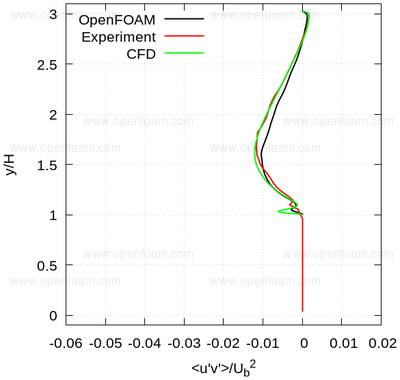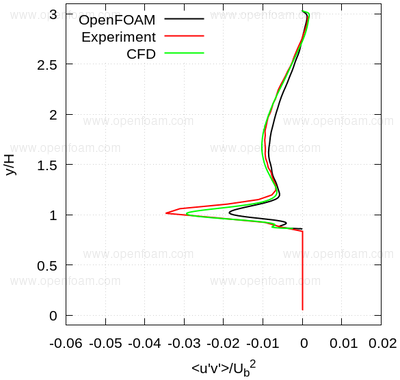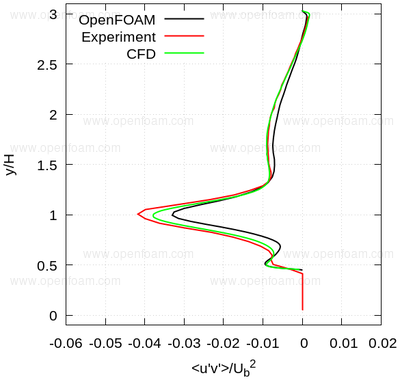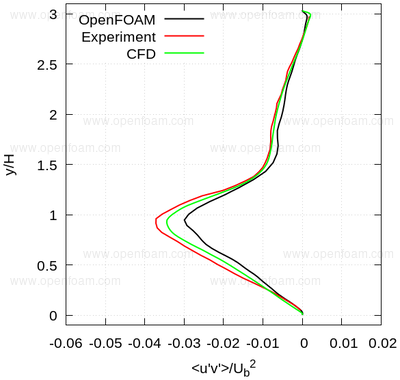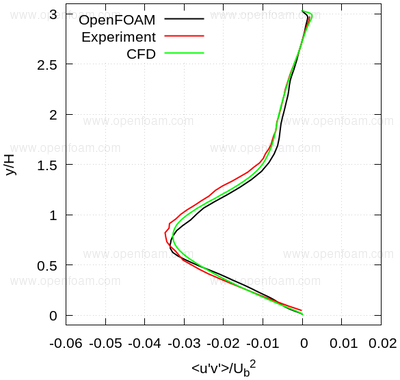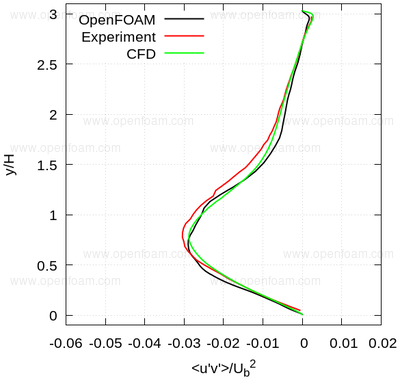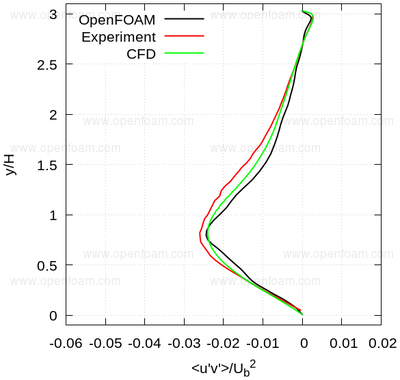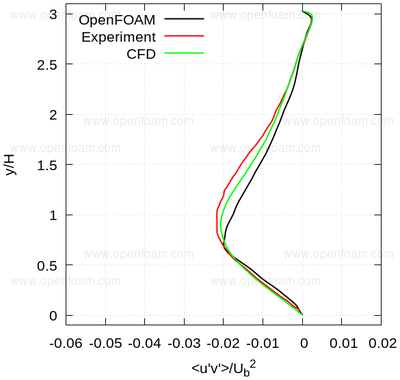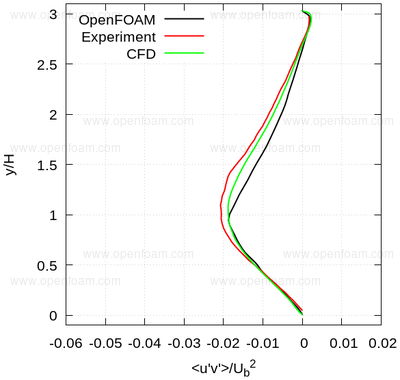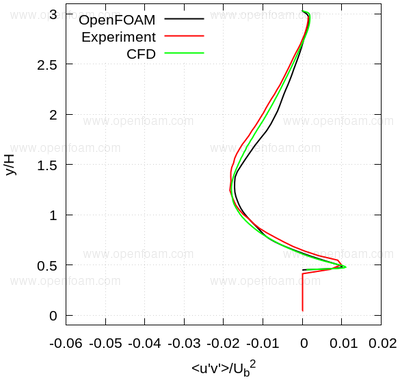## Overview🔗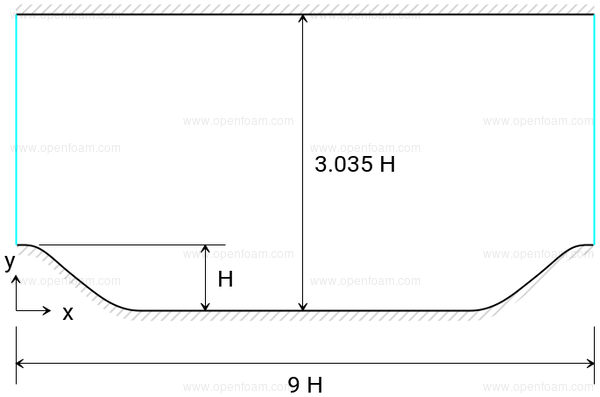## Mesh🔗

• 3D structured mesh created using blockMesh
• hills described by the function

$y(x) = \begin{cases} \min(1, a_1 + b_1 x + c_1 x^2 + d_1 x^3) & 0 \le x \lt 9, \\ a_2 + b_2 x + c_2 x^2 + d_2 x^3 & 9 \le x \lt 14, \\ a_3 + b_3 x + c_3 x^2 + d_3 x^3 & 14 \le x \lt 20, \\ a_4 + b_4 x + c_4 x^2 + d_4 x^3 & 20 \le x \lt 30, \\ a_5 + b_5 x + c_5 x^2 + d_5 x^3 & 30 \le x \lt 40, \\ \max(0, a_6 + b_6 x + c_6 x^2 + d_6 x^3) & 40 \le x \lt 54. \\ \end{cases}$
a b c d
1 $$28$$ $$0$$ $$6.775070969851 \times 10^{-3}$$ $$- 2.124527775800 \times 10^{-3}$$
2 $$25.07355893131 \times 10^0$$ $$0.9754803562315 \times 10^{0}$$ $$- 1.016116352781 \times 10^{-1}$$ $$1.889794677828 \times 10^{-3}$$
3 $$2.579601052357 \times 10^1$$ $$8.206693007457 \times 10^{-1}$$ $$- 9.055370274339 \times 10^{-2}$$ $$1.626510569859 \times 10^{-3}$$
4 $$4.046435022819 \times 10^1$$ $$-1.379581654948 \times 10^{0}$$ $$1.945884504128 \times 10^{-2}$$ $$- 2.070318932190 \times 10^{-4}$$
5 $$1.792461334664 \times 10^1$$ $$8.743920332081 \times 10^{-1}$$ $$- 5.567361123058 \times 10^{-2}$$ $$6.277731764683 \times 10^{-4}$$
6 $$5.639011190988 \times 10^1$$ $$-2.010520359035 \times 10^{0}$$ $$1.644919857549 \times 10^{-2}$$ $$2.674976141766 \times 10^{-5}$$
• this has been set in the blockMeshDict using a codeStream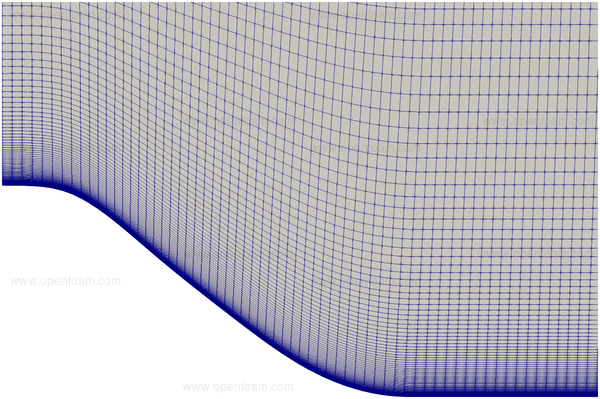## Boundary conditions🔗

• The mean bulk velocity $$\u_b$$ at the inlet patch is defined as:

$\u_b = \frac{1}{2.0355H}\int\limits_{H}^{3.035H} \u_x (y) dy$
• This is set to 1 m/s, and maintained using a mean velocity force fvOption
• The laminar viscosity is set to achieve the target Reynolds numbers, where the reference length scale is given by the hill height

• The laminar viscosity is derived from the Reynolds number, i.e.

$\nu_\infty = \frac{|\u_b| H}{Re} = \frac{1 \times 0.028}{10565} = 2.65 \times 10^{-6} m^2/s$

### Common fields🔗

Velocity: U

Patch Condition Value
Inlet cyclic
Outlet cyclic
Hills noSlip
Walls noSlip

Pressure: p

Patch Condition Value
Inlet cyclic
Outlet cyclic

### Turbulence fields🔗

Turbulence viscosity: nut

Patch Condition Value
Inlet cyclic
Outlet cyclic
Hills nutUSpaldingWallFunction
Walls nutUSpaldingWallFunction

#### Spalart-Allmaras IDDES

Modified turbulence viscosity: nuTilda

Patch Condition Value
Inlet cyclic
Outlet cyclic
Hills fixedValue 0
Walls fixedValue 0

## Results🔗

The precursor steady computation is used to initialise the transient calculation. After evolving the transient case for XXX flow-throughs a fully turbulent flow is established, as shown by the instantaneous velocity:The average velocity prediction shows differences compared to the velocity derived from the precursor steady calculation: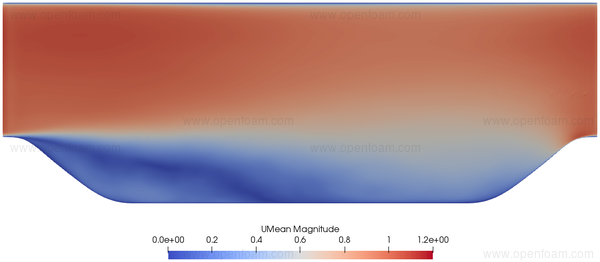Turbulent structures are clearly evident in the instantaneous Q criterion prediction: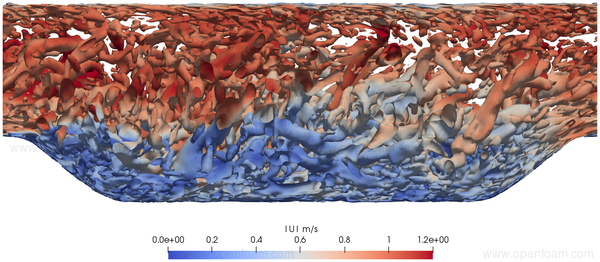The following series of images provide a quantitative comparison between OpenFOAM predictions and both measured data and results from another CFD code at various streamwise locations.

### Profiles🔗

Average velocity profiles: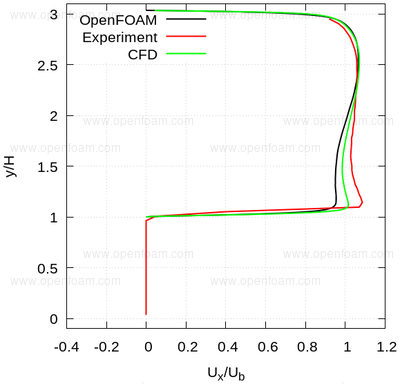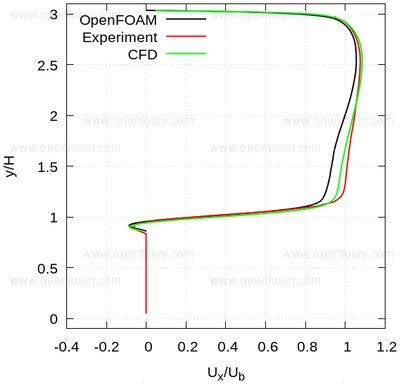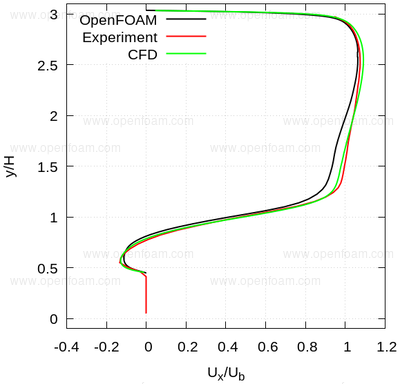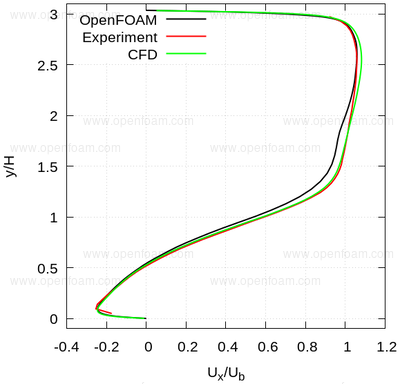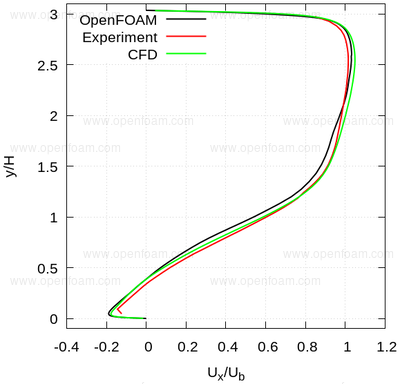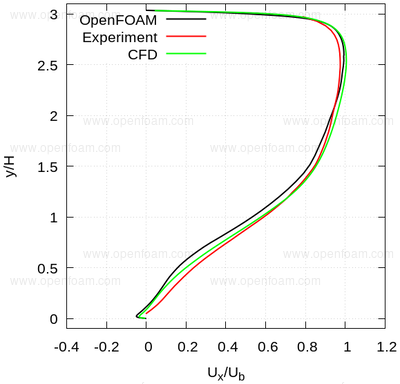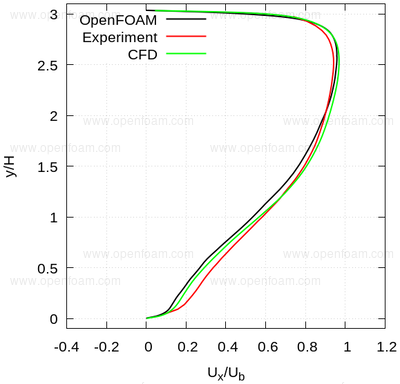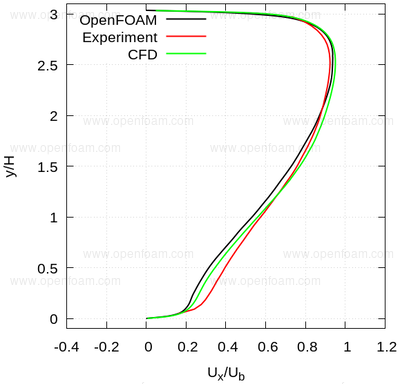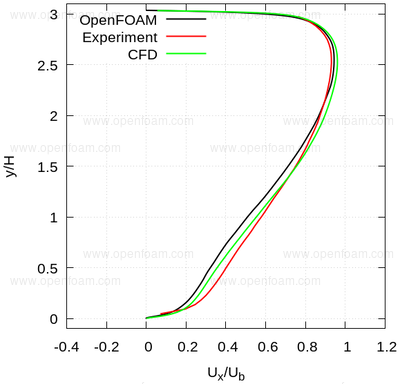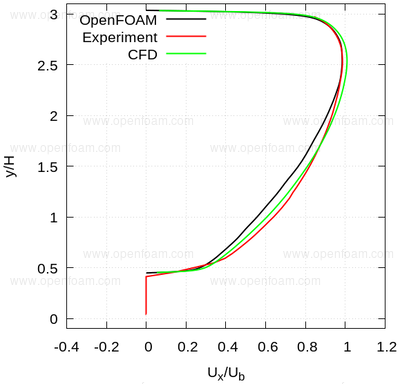Average normal stresses: uu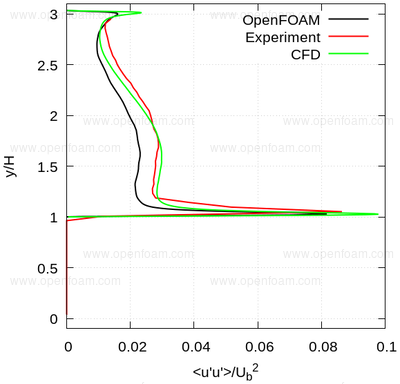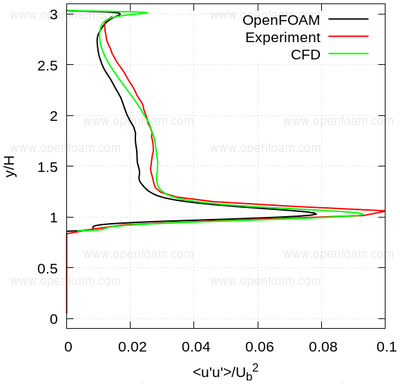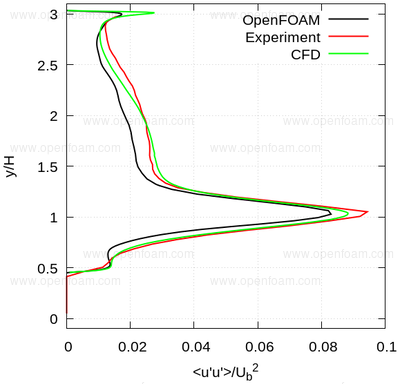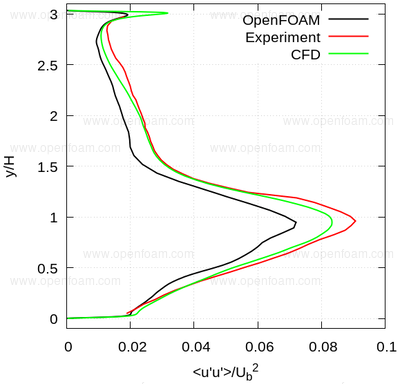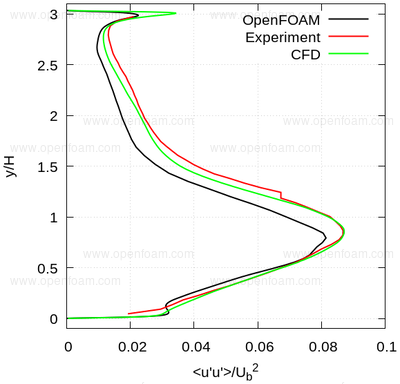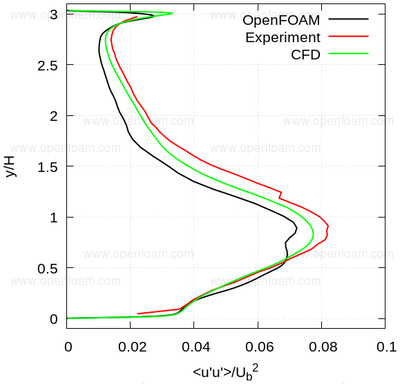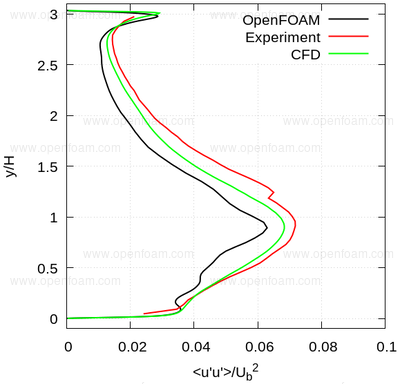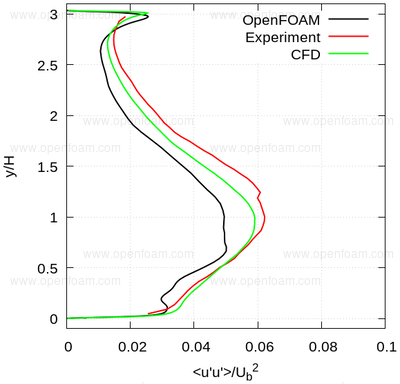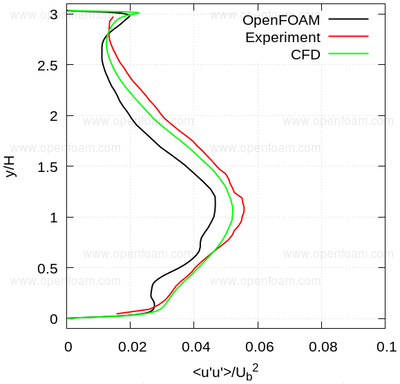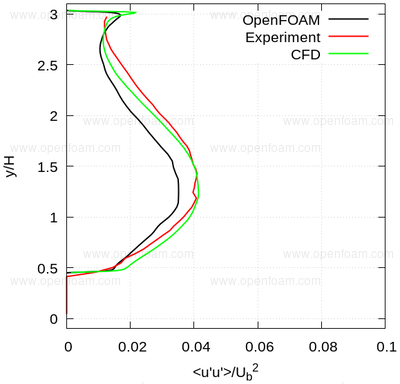Average normal stresses: vv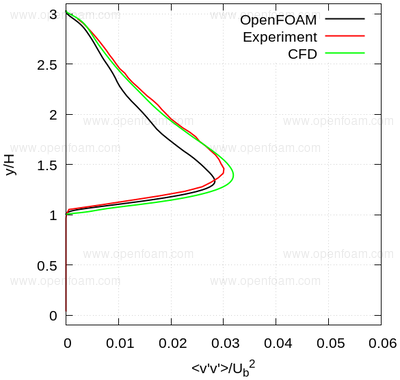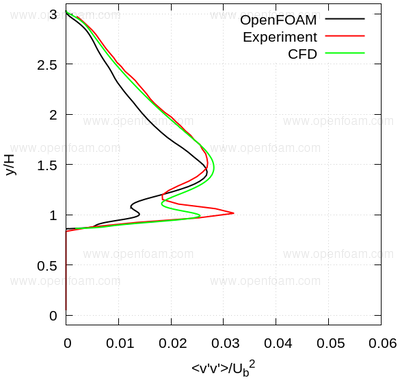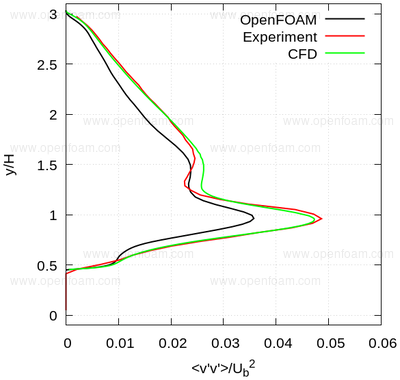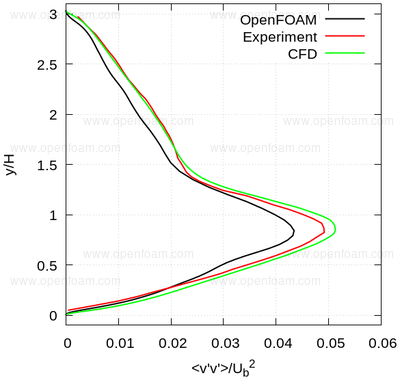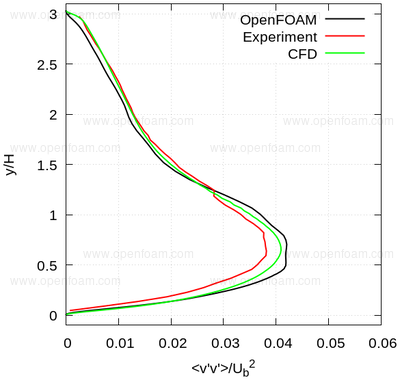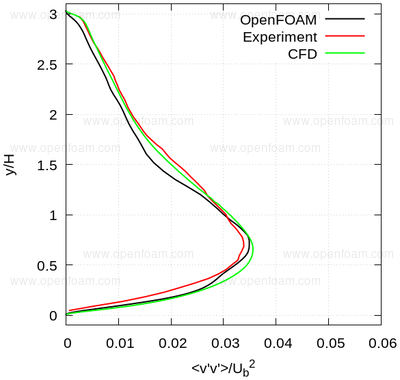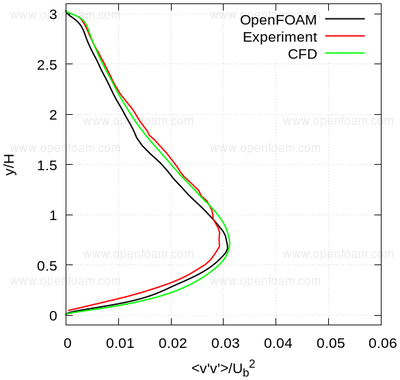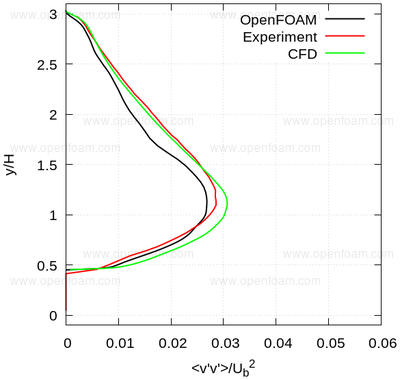Average shear stress: uv